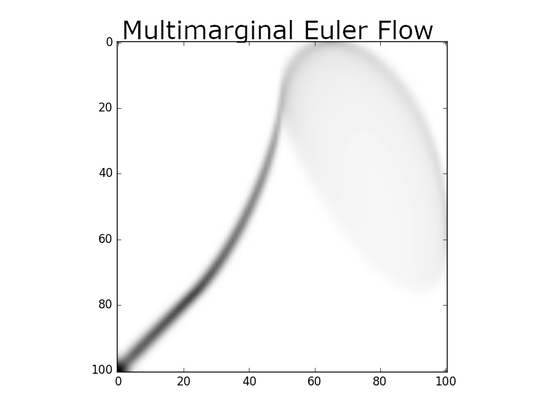# Projects

#### Conference: New Monge Problems and Applications

A two days conference centered on computational optimal transport and its applications, September 14th-15th, University Gustave Eiffel, …#### Séances du groupe de travail

Page du groupe de travail du labex Bézout sur les problèmes de transport optimal: Transport martingale, transport optimal faible, …

# Posts

### Beyond Sinkhorn: Generalized Iterative Scaling

Sinkhorn algorithm (also called IPFP for Iterative Proportional Fitting Procedure) is an alternating optimisation algorithm which has …

### A geometric formulation of Laplace method.

When trying to understand the low temperature asymptotic of entropic regularization in optimal transport, Gibbs measures naturally …

### Partial optimization and Schur complement

In a couple of recent papers on applied optimal transport (OT), we used the fact that the so-called semi-dual formulation of OT …

In a project with a student, I recently derived a non-local diffusion PDE which turns out, surprisingly for me, to have a name: Stein …

# Recent preprints and publications

Complete list of publications here.

### Inverse Consistency by Construction for Multistep Deep Registration

A multistep deep registration framework that produces inverse consistent registration.

### A geometric Laplace method

We give a geometric formulation of the first-order term of the Laplace method in a particular case. Our main result expresses the …

### Unbalanced Optimal Transport, from Theory to Numerics

An easy to read review of extension of optimal transport to positive measures, with an emphasis on numerics and entropic …

### On the existence of Monge maps for the Gromov-Wasserstein distance

In Euclidean space, we prove existence of optimal Monge maps for the case of the inner product cost and for the case of the quadratic …

Learning with gradient inverse consistency and no explicit regularization achieves better diffeomorphic registration performances than …

### Stability of Semi-Dual Unbalanced Optimal Transport: fast statistical rates and convergent algorithm

We derive stability estimates on the semi-dual formulation of unbalanced optimal transport and propose an optimization algorithm, …

# Talks

### On the Gromov-Wasserstein problem, existence of Monge maps and Unbalanced Gromov-Wasserstein

This talk has two parts. First we present a possible extension of the Gromov-Wasserstein problem to the setting of metric measures spaces, whose total mass is not necessarily equal to 1. We propose a true distance and a lower bound which is more friendly for computations. Second, we study the existence of Monge maps as optimizer of the standard Gromov-Wasserstein problem for two different costs in euclidean spaces. The first cost for which we show existence of Monge maps is the scalar product, the second cost is the quadratic cost between the squared distances for which we show the structure of a bi-map. We present numerical evidence that the last result is sharp.

### On the Gromov-Wasserstein problem, existence of Monge maps and Unbalanced Gromov-Wasserstein

This talk has two parts. First we present a possible extension of the Gromov-Wasserstein problem to the setting of metric measures spaces, whose total mass is not necessarily equal to 1. We propose a true distance and a lower bound which is more friendly for computations. Second, we study the existence of Monge maps as optimizer of the standard Gromov-Wasserstein problem for two different costs in euclidean spaces. The first cost for which we show existence of Monge maps is the scalar product, the second cost is the quadratic cost between the squared distances for which we show the structure of a bi-map. We present numerical evidence that the last result is sharp.

### Statistical estimation of optimal transport potentials

We show how to break the curse of dimension for the estimation of optimal transport distance between two smooth distributions for the Euclidean squared distance. The approach relies on essentially one tool: represent inequality constraints in the dual formulation of OT by equality constraints with a sum of squares in reproducing kernel Hilbert space. By showing this representation is tight in the variational formulation, one can then leverage smoothness to break the curse. (*) However, the constants associated with the algorithm a priori scale exponentially with the dimension.

# Experience

#### Université Gustave Eiffel

Sep 2018 – Present Noisy-Champs
Member of the research lab LIGM (Laboratoire d’informatique Gaspard Monge) and teaching signal processing.

#### University Paris-Dauphine

Sep 2011 – Sep 2018 Paris
Member of the research lab Ceremade, UMR CNRS 7534, and teaching applied mathematics.

#### Imperial College

May 2009 – Sep 2011 London
Member of the Institute for Mathematical Sciences and the Math department.

#### ENS Cachan (ENS Paris-Saclay)

Sep 2005 – May 2009 Paris area
Member of the lab CMLA.

# PhD and HDR

#### Habilitation à diriger les recherches

Optimal transport, Diffeomorphisms and applications to imaging

#### PhD in applied mathematics

Hamiltonian formulation of diffeomorphic image matching